Zurück zu meiner Homepage

# Statistical Tables Part 2 / Part 1

 Copyright by Dr. Achim Lewandowski (homepage), Erlangen

## Part 2: Discrete Distributions

 Binomial Negative-Binomial Geometric Poisson Hypergeometric

## Help: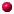Very important: Your browser must understand JavaScript! Try Netscape 3.0.It happens sometimes that pressing the buttons has no effect at all. This seems to be a bug of the JavaScript-interpreter. (With Netscape 3.0 I have not observed this error yet!). Try to reload this document. Or try to switch to part 1 and back to part 2.There are two parts: Part 1 (Continuous distribution functions) enables you to calculate cumulative distribution functions and their inverse functions. For discrete random variables (Part 2, this page) you enjoy the possibility to calculate probability and cumulative probability functions.Almost all algorithms were converted from Turbo Pascal to JavaScript (Source: Numerical Recipes in Pascal). The books of Johnson+Kotz (Continuous Distributions I+II, Discrete Distributions) were also used.You must use the decimal point!As input simple numbers but also mathematical more complex expressions are allowed. For example the input of Mu=1 and Sigma=1 for the normal distribution gives the same result as Mu=3*Math.pow(4,1/2)-5 and Sigma=2*Math.sin(Math.PI/2)-1 or Mu=3-2 and Sigma=-1+2*1.You are allowed to copy the necessary files (zipped in tables_e.zip (29 k). Please do not change anything and always indicate the source.
Known bugs (and restrictions):The computation of the inverse of the F-distribution sometimes fails!The algorithms of the inverse of the Gamma and the Chi-Square-distribution are quite slow, so please wait for a few seconds!The cumulative distribution function of the Hypergeometric distribution is calculated exactly (simple additions), that's why I've restricted the computation: If more than 100 additions have to be done, the calculation is interrupted!For n>2000 the normal distribution is used instead of the t-distribution.Don't try to type in something like "@#%", only an error will occur (and no usable result). So please concentrate and don't try any tricks!I've tried my best, but I give no warranty that the algorithms work correctly under all circumstances. If you are sure to have recognised an error, please send me an e-mail. Tell me the name of the distribution and the values of the parameters that have produced the wrong result.I don't give any warranty for the correctness of all calculations! An advice: if you need to calculate F(x) out of x, so calculate also the inverse function of F(x), then the original value x should appear. With Copy and Paste this is done in a few seconds !

 The statistical tables have been used in the following articles:"Calorie restriction extends Saccharomyces cerevisiae lifespan by increasing respiration," Su-Ju Lin, Matt Kaeberlein, Alex A. Andalis, Lori A. Sturtz, Pierre-Antoine Defossez, Valeria C. Culotta, Gerald R. Fink & Leonard Guarente, NATURE | VOL 418 | 18 JULY 2002"Analysis of a Mutant Histone H3 That Perturbs the Association of Swi/Snf with Chromatin", Andrea A. Duina and Fred Winston, MOLECULAR AND CELLULAR BIOLOGY, Vol. 24, No. 2, p. 561-572 (Jan. 2004)"Saccharomyces cerevisiae SSD1-V Confers Longevity by a Sir2p-Independent Mechanism", Matt Kaeberlein, Alex A. Andalis, Gregory B. Liszt, Gerald R. Fink and Leonard Guarente, Genetics 166: 1661-1672 (April 2004)"Defects Arising From Whole-Genome Duplications in Saccharomyces cerevisiae", Alex A. Andalis, Zuzana Storchova, Cora Styles, Timothy Galitski, David Pellman and Gerald R. Fink, Genetics 167: 1109-1121 ( July 2004)"Coordinated Regulation of Growth Genes in Saccharomyces cerevisiae", Matthew G. Slattery and Warren Heideman, Cell Cycle 6:10, 1210-1219, 15 May 2007; �2007 Landes Bioscience"Protein Kinase A, TOR, and Glucose Transport Control the Response to Nutrient Repletion in Saccharomyces cerevisiae", Matthew G. Slattery, Dritan Liko, and Warren Heideman, Eukaryotic Cell | VOL 7 | Nr 2 | February 2008, 358-367"Nutrient regulation of cell growth and cell proliferation in Saccharomyces cerevisiae", Matthew Grant Slattery, Thesis, 2008."Extensive role of the general regulatory factors, Abf1 and Rap1, in determining genome-wide chromatin structure in budding yeast", Mythily Ganapathi, Michael J. Palumbo, Suraiya A. Ansari, Qiye He, Kyle Tsui, Corey Nislow, and Randall H. Morse, Nucleic Acids Research, 2010, 1-13"Maintenance of Open Chromatin States by Histone H3 Eviction and H2A.Z", Laura Marie Lombardi, Dissertation, eScholarship University of California 2011

 Binomial Negative-Binomial Geometric Poisson Hypergeometric

### Binomial Distribution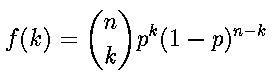n = p= k = f(k) = F(k) =
 Binomial Negative-Binomial Geometric Poisson Hypergeometric

### Negative-Binomial-Distribution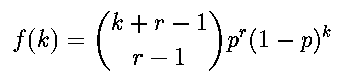r = p= k = f(k) = F(k) =
 Binomial Negative-Binomial Geometric Poisson Hypergeometric

### Geometric Distribution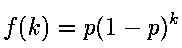p = k = f(k) = F(k) =
 Binomial Negative-Binomial Geometric Poisson Hypergeometric

### Poisson DistributionLambda = k = f(k) = F(k) =
 Binomial Negative-Binomial Geometric Poisson Hypergeometric

### Hypergeometric Distribution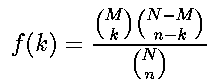M = N= n = k = f(k) = F(k) =
 Binomial Negative-Binomial Geometric Poisson Hypergeometric